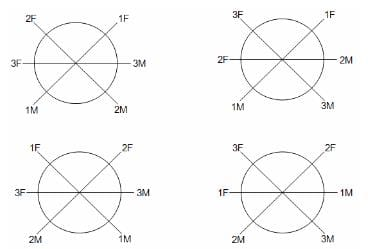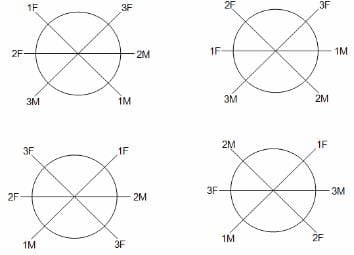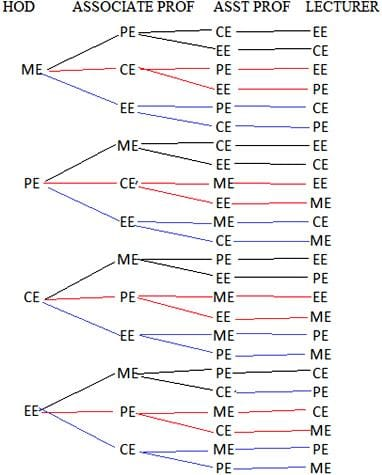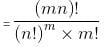CAT  >  Test Level 3: Permutation & Combination - 2

# Test Level 3: Permutation & Combination - 2

Test Description

## 10 Questions MCQ Test Level-wise Tests for CAT | Test Level 3: Permutation & Combination - 2

Test Level 3: Permutation & Combination - 2 for CAT 2023 is part of Level-wise Tests for CAT preparation. The Test Level 3: Permutation & Combination - 2 questions and answers have been prepared according to the CAT exam syllabus.The Test Level 3: Permutation & Combination - 2 MCQs are made for CAT 2023 Exam. Find important definitions, questions, notes, meanings, examples, exercises, MCQs and online tests for Test Level 3: Permutation & Combination - 2 below.
Solutions of Test Level 3: Permutation & Combination - 2 questions in English are available as part of our Level-wise Tests for CAT for CAT & Test Level 3: Permutation & Combination - 2 solutions in Hindi for Level-wise Tests for CAT course. Download more important topics, notes, lectures and mock test series for CAT Exam by signing up for free. Attempt Test Level 3: Permutation & Combination - 2 | 10 questions in 20 minutes | Mock test for CAT preparation | Free important questions MCQ to study Level-wise Tests for CAT for CAT Exam | Download free PDF with solutions
 1 Crore+ students have signed up on EduRev. Have you?
Test Level 3: Permutation & Combination - 2 - Question 1

### A married couple invites two other married couples for a round-table dinner party. In how many ways can they be seated if each one sits diametrically opposite to his/her spouse?

Detailed Solution for Test Level 3: Permutation & Combination - 2 - Question 1

The first possibility is that all the three males are sitting together.
In this arrangement ,all the females will then be sitting together opposite their husbands.
Number of ways = 3 x 2 = 6.
The 2nd possibility is that a male and a female are sitting alternately.
There are 2 such ways.
The number of ways is as shown in the diagrams below.Test Level 3: Permutation & Combination - 2 - Question 2

### There are 3 ways from a town A to reach town B, 4 ways from town B to reach town C, and 2 ways from town A to reach town C directly. In how many ways can a person go from town A to town C and then return to A, if he does not take the way already taken?

Detailed Solution for Test Level 3: Permutation & Combination - 2 - Question 2

Case i:
Person goes directly:
Number of ways = 2
If he returns either directly without taking the same path or through B, then number of ways = 1 + 3 x 4 = 13
Total number of ways = 2 x 13 = 26

Case ii:
Person goes through B:
Number of ways = 3 x 4 = 12
If he returns either directly or through B without taking the same path, then number of ways = 2 + 2 x 3 = 8
Total number of ways = 12 x 8 = 96
Hence, total number of ways in both cases = 26 + 96 = 122

Test Level 3: Permutation & Combination - 2 - Question 3

### Four departments in an engineering college (ME, PE, CE and EE) contain 4 faculty members each, i.e. an HOD, an Associate Professor, an Assistant Professor and a Lecturer. Each department also has 5 student coordinators. A panel of 4 members is to be constituted for a conference such that 1 faculty member is selected from each department, so that the final committee has an HOD, an Associate Professor, an Assistant Professor and a Lecturer. Also, 10 student coordinators have to be selected and no restrictions exist for the selection of the student coordinators. In how many ways can the panel be constituted including the selection of the student coordinators?

Detailed Solution for Test Level 3: Permutation & Combination - 2 - Question 3

An HOD can be selected in four ways. Further, an Associate Professor can be selected in three ways, then an Assistant Professor can be selected in 2 ways and then there is only one way for the selection of a Lecturer.
Number of ways of selecting the faculty members = 4 × 3 × 2 × 1 = 4! = 24For selection of student coordinators, the number of ways is 20C10.
Therefore, total number of ways to select a panel including the student coordinators = 4! × 20C10

Test Level 3: Permutation & Combination - 2 - Question 4

From the 7 HODs of seven departments of an engineering college, one is to be promoted to be the principal of the college. Further, the position which is now vacant for the HOD is to be filled by an Associate Professor being promoted as an HOD. And further, one Assistant Professor is to be promoted to be an Associate Professor. No further promotions are made. The number of Associate Professors in the college is 4, 5, 3, 5, 2, 1 and 4 and that of Assistant Professors is 8, 9, 12, 5, 6, 7 and 6 for the departments of Mechanical, Electrical, Electronics, Civil, Chemical, Computer Science and Production Engineering, respectively. In how many different ways can the changes occur?

Detailed Solution for Test Level 3: Permutation & Combination - 2 - Question 4

If the HOD of Mechanical department is promoted to be the principal, the number of possibilities for the new HOD is 4 and the number of possibilities for the new Associate Professor is 8.
So, the total number of possibilities of changes in this case is 4 × 8 = 32.
Similarly, for Electrical department, Number of cases = 5 × 9 = 45
For Electronics department, Number of cases = 3 × 12 = 36
For Civil department, Number of cases = 5 × 5 = 25
For Chemical department, Number of cases = 2 × 6 = 12
For Computer Science department, Number of cases = 1× 7 = 7
For Production department, Number of cases = 4 × 6 = 24
Therefore, Total number of changes possible = 32 + 45 + 36 + 25 + 12 + 7 + 24 = 181

Test Level 3: Permutation & Combination - 2 - Question 5

In a game, a person has to select three cards from a deck of cards. If he selects a face card, then the other two cards also have to be face cards. If a number card is selected, then all the other cards have to of the same number. In how many different ways can the selection be made?

Detailed Solution for Test Level 3: Permutation & Combination - 2 - Question 5

First Case: All three cards are face cards; Number of possible cases = 12C3
Second Case: First, a number has to be selected; this can be done in 9 ways. Now, three cards of this number have to be selected, which can be done in 4C3 ways.
Therefore, number of cases = 9 × 4C3
Therefore, total number of cases = 12C3 + 9 × 4C3

Test Level 3: Permutation & Combination - 2 - Question 6

On a plane paper, 11 dots are marked. Out of these dots, only 5 lie in a straight line. Rest of them are haphazardly spread out throughout the paper. How many unique triangles can be formed by joining them?

Detailed Solution for Test Level 3: Permutation & Combination - 2 - Question 6

A triangle can be formed by joining 3 non-linear dots, so 11C3 triangles would have been formed if no dots were in a straight line. But 5 dots, which are in a straight line, instead of giving 5C3 triangles, gives no triangle. Therefore, required number of triangles = 11C35C3 = (11 x 10 x 9 x 8!/(3!8!)) – (5 x 4 x 3!/(3!2!)) = 165 – 10 = 155

Test Level 3: Permutation & Combination - 2 - Question 7

Detailed Solution for Test Level 3: Permutation & Combination - 2 - Question 7

Case i: All of same colour WWW, BBB, RRR
In this case, total number of necklaces = 3
Case ii: 2 of same colour and 1 of other colour.
2 of same colour and 1 unique will have 6 cases.
These can be arranged in 6 × 3 = 18
Case iii: All different coloured beads in a necklace
The arrangements WBR, WRB, BRW, BWR, RWB and RBW will be considered as only one arrangement.
In this case, total number of necklaces = 1
So, total number of necklaces = 3 + 18 + 1 = 22

Test Level 3: Permutation & Combination - 2 - Question 8

A married couple invites 6 other couples for a dinner party. They are seated around a long table with one seat each at the short ends of the table and 6 seats each at either long side. In how many ways can they be seated if the hosts sit at the short ends and the other guests are free to sit anywhere?

Detailed Solution for Test Level 3: Permutation & Combination - 2 - Question 8

The hosts can take the seats at the short ends in 2! ways.
Note that the number of ways in which mn different items can be divided equally into m groups, each containing n objects and where the order of the groups is not important is given by:
Number of ways12 guests can be divided into 2 groups of 6 each in (12!)/[(6!)2 (2!)] ways, (m = 2, n = 6).
They can then be assigned to either side of the long table in 2! x (12!)/[(6!)2 (2!)] = (12!)/(6!)2 ways.
Next on each side, 6 people can sit in 6! ways.
Hence, total number of ways = 2! x (6!)2 x (12!)/(6!)2 = 2(12!)

Test Level 3: Permutation & Combination - 2 - Question 9

The number of words formed by arranging all the letters in the word EDUCATION, such that the letters CAT appear in the same place as in the given word and no vowels come together, is

Detailed Solution for Test Level 3: Permutation & Combination - 2 - Question 9

Since the letters CAT appear in the same place as in the word EDUCATION, the numbers of vowels (E, U, I, O) and consonants (D, N) that we need to arrange are 4 and 2, respectively.
Since no vowels are supposed to come together, the vowels can take 1st, 3rd, 7th or 9th places and the remaining places can be filled by consonants.
So, the required number of ways = 4! x 2! = 48

Test Level 3: Permutation & Combination - 2 - Question 10

If w represents the cube root of unity, then the number of values of (p, q, r), such that wp + wq + wr = 0, where p, q and r are positive integers less than 9, is

Detailed Solution for Test Level 3: Permutation & Combination - 2 - Question 10

p, q and r can take values 1, 2, 3, ..., 8.
We know that 1 + w + w2 = 0, w3 = w6 = 1, w2 = w5 = w8 and w = w4 = w7.
Thus, a selection has to be made by choosing exactly one digit from each of the brackets: (3, 6), (2, 5, 8) and (1, 4, 7).
This can be done in 2 x 3 x 3 = 18 ways.

## Level-wise Tests for CAT

5 docs|272 tests
 Use Code STAYHOME200 and get INR 200 additional OFF Use Coupon Code
Information about Test Level 3: Permutation & Combination - 2 Page
In this test you can find the Exam questions for Test Level 3: Permutation & Combination - 2 solved & explained in the simplest way possible. Besides giving Questions and answers for Test Level 3: Permutation & Combination - 2, EduRev gives you an ample number of Online tests for practice

## Level-wise Tests for CAT

5 docs|272 tests

### How to Prepare for CAT

Read our guide to prepare for CAT which is created by Toppers & the best Teachers